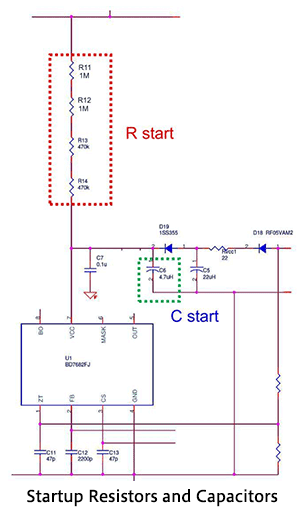# Selecting Critical Components: VCC-Related Components of Power Supply ICs

2018.12.20

・The IC power supply VCC is generated by the VCC windings using the secondary-side output.

・Upon startup, a secondary-side output is not generated, and so a circuit to supply a voltage for startup is provided separately.

・In order to avoid erroneous VCC OCP activation, a resistor to limit the VCC windings surge voltage is necessary.

In this article, we determine component values related to the VCC pin of the power supply IC used in the design. The VCC pin is the power supply pin of the BD7682FJ power supply IC.

The internal control circuits of the BD7682FJ operate under the voltage applied to the VCC pin. As a matter of common sense, not only is direct operation not possible when a voltage in the range 300 V to 900 V is input to the power supply circuit, but the device would probably fail instantaneously. In order to use this power supply IC, a low DC voltage must be generated. The VCC operating voltage range is from 15.0 to 27.5 V, and as already explained in “ Transformer T1 Design – 2”, when calculating the transformer VCC windings Nd (also called the auxiliary windings or the tertiary windings), Nd was calculated assuming VCC = 24 V.

The circuit diagram on the right is an excerpt of the relevant section. Here we shall determine the component values for the circuit areas related to “VCC voltage generation” (within the orange box), “VCC windings surge voltage limiting” (within the orange box) and “VCC startup” (within the blue box).

## Rectifying Diode D18 and Smoothing Capacitor C5 for VCC Voltage Generation

The switching voltage occurring across the VCC windings Nd (windings 5-6 in the circuit diagram) is rectified to a DC voltage and smoothed by the diode D18 and capacitor C5 within the orange box in the circuit diagram. This circuit is the same as a simple diode-rectifying DC-DC converter. (The inductor L4 included within the box is not actually used, and should be ignored. The resistor Rvcc1 is a surge limiting resistor, and is explained later.)

The rated voltage of D18 is determined by calculating the reverse voltage applied to D18.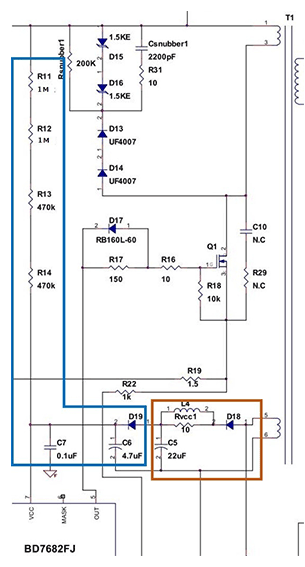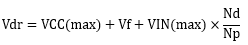We set VCC(max) at 31.5 V. The VCC pin has a VCC OVP (overvoltage protection) function, with a maximum value of 31.5 V, so that even should the VCC voltage rise to this voltage, the D18 rated voltage would not be exceeded. Vf is set to 1 V, and VIN(max) is 900 V. From the results obtained in “Transformer T1 Design – 2”, Nd is set to 8 turns, and Np is similarly set to 64 turns.

Substituting these values, we have: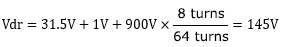As a value that includes a margin, we use 145 V/0.7 ? 200 V, and so we select a diode with a rated voltage of 200 V. In view of the objectives, D18 must be a diode type that is appropriate for high speed switching. Here we use the ROHM fast-recovery diode RF05VAM2S.

As the capacitor C5, a 22 μF aluminum electrolytic capacitor is appropriate, with the rated voltage set to 35 V given the value of Vcc(max).

## Surge Voltage Limiting Resistor Rvcc1 for VCC Windings

Due to the transformer leakage inductance (Lleak), a large surge voltage (spike noise) occurs at the moment when the MOSFET is switched from on to off. This surge voltage is induced in the VCC windings, and there is the possibility that the VCC voltage may rise to activate VCC OVP at the VCC pin. In order to reduce this surge voltage, a limiting resistor Rvcc1 of about 5 to 22 Ω is inserted. In actuality, the increase in VCC voltage should be checked with the circuit incorporated into the product, and the resistance value adjusted.

## Resistors R11, R12, R13, R14, Capacitor C6, Diode D19 for VCC Startup

The VCC voltage resulting from the VCC windings is based on the secondary-side output (Ns:Nd). Hence in principle, if the circuit does not begin switching operation, a VCC voltage does not appear, and so at startup the VCC voltage must be applied separately to the IC. The startup resistors (Rstart) R11, R12, R13, R14, together with the startup capacitor (Cstart) C6, start up the IC. This CR circuit is also used to adjust the startup time. Apart from this, power consumption during standby is also affected.

The startup resistors Rstart are determined from the minimum and maximum conditions indicated by the equations below. VIN_start is set to 180 V, adding a margin to VIN_min. VCC UVLO(max) is 20 V as given by the data sheet, and the standby circuit current IOFF, that is, the VCC current before startup is at maximum 30 μA, also from the data sheet, but adding a margin, 40 μA is used. VCC OVP(max) is 31.5 V from the data sheet, and the VCC current during protection circuit operation Ion1 is set to the minimum value of 300 μA.

Rstart＜VIN_start－VCC UVLO（max）/IOFF＝（180V－20V）/40μA＝4000kΩ

Rstart＞VIN_max－VCC OVP（max）/Ion1＝（900V－31.5V）/300μA＝2895kΩ

2895kΩ＜Rstart＜4000kΩ

Using these results, Rstart is set to 2940 kΩ (1 MΩ each for R11 and R12, 470 kΩ each for R13 and R14).

The startup capacitor (Cstart) C6 also serves to stabilize VCC, and so a value of 2.2 μF or higher is recommended. Here, partly in consideration of the startup time described above, a value of 4.7 μF is used. The graph shows the relation between VIN and the startup time for different Cstart values.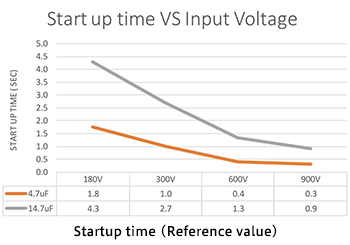Regarding the relationship with the startup resistance Rstart, if a low value is set for Rstart the startup time is short, and the standby power is high. Conversely, if Rstart is set to be high, the startup time is long, and the standby power is low.

When VIN is input, C6 is charged, and when the voltage at the VCC pin reaches the startup voltage, IC operation begins. Thereafter, when the output voltage exceeds a constant voltage, the VCC generation circuit operates to supply the VCC voltage. The diode D19 acts to prevent the smoothing capacitor C5 from being charged during startup. As the diode D19, the switching diode 1SS355VM (manufactured by ROHM), with a small reverse current IR, is used. These circuit diagram should also be studied (in this circuit, Rvcc is 22 Ω).